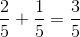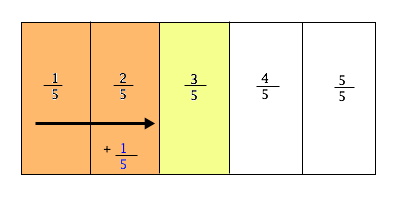# SSAT Middle Level Math : How to add fractions

## Example Questions

1 2 15 16 17 18 19 20 21 23 Next →

### Example Question #221 : How To Add Fractions

Solve: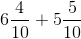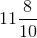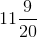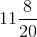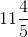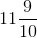Explanation:

When we add mixed numbers, we add whole numbers to whole numbers and fractions to fractions.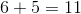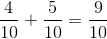Remember, when we are adding fractions we must have common denominators and we only add the numerators.

### Example Question #65 : Fractions

On Monday it snowedof an inch in the afternoon andof an inch in the evening. What was the total amount of snowfall on Monday?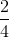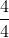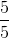Explanation:

To solve this problem, we are putting the amount of snowfall from the afternoon and the evening together, so we add the fractions.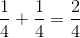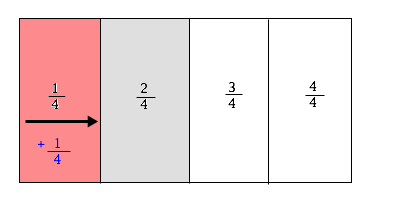### Example Question #13 : Express A Fraction With Denominator 10 As An Equivalent Fraction With Denominator 100: Ccss.Math.Content.4.Nf.C.5

Solve the following: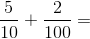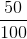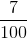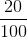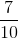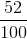Explanation:

When we add fractions, we must have common denominators. Whenever we have a number overorwe can add(s) to the numerator and denominator to make common denominators.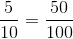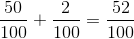### Example Question #1 : Build Fractions From Unit Fractionsis equal to which of the options below?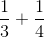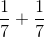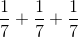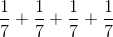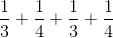Explanation:

When we add fractions, we have to have common denominators and we only add the numerators.### Example Question #1 : Adding Fractions In Word Problems

In Charlie's pantry,of the items are potato chips,of the items are tortilla chips, and the rest are cookies or crackers. What fraction are chips?